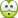# Lagrangian of electromagnetism

• I
hi, I would like to put into words that I really wonder how these lagrangian or lagrangian densities are created. For instance in the link at 59.35 suskind says $$\int A^u dx^u$$ is invariant or action integral. How is this possible ???Could you provide me with the proof???

## Answers and Replies

21st century video presentations from higher education sources lack the attributes of higher teaching.
(Say what? ;)
If the student hasn't learned. The teacher hasn't taught. Seek out an alternative presentation.
Increase your knowledge base sampling rate by relying upon multiple sources for what you're striving to learn.

John

you want to say: Is suskind saying something wrong in this topic ????

ıs there anyone who is capable of giving some answers to my question with a mathematical demonstration????? I am really eager to see it, and looking forward to your responses....

Guys, It has been 2 days, and I can not get a satisfying answer to my question, I really wonder your valuable responses....

jtbell
Mentor
It has been 2 days
According to my arithmetic, it was only one day and two hours, when you posted this.I'll move this over to the Classical Physics forum to see if it draws more useful responses. General Physics is for introductory-physics-type questions.

Dale
Mentor
2020 Award
I can not get a satisfying answer to my question
I read your question and watched the video and I am not sure what you are asking. Could you clarify?

In particular, are you asking how a quantity like ##A_u dx^u## is invariant, or are you asking how it can be integrated, or are you asking something else?

ChrisVer
Gold Member
How is that integral invariant:
Make a Lorentz transformation and you get the answer, I mean common, it's a SR course... in fact it's a scalar quantity by construction I'd say, since it's the Minkowski inner product. It will get you to an equation like $\eta_{\mu\nu} \Lambda^\mu_\sigma \Lambda^\nu_\rho$ which is equal to $\eta_{\rho \sigma}$.
https://en.wikipedia.org/wiki/Special_relativity#Metric

If you want another example not from the SR but from classical mechanics, why is the $|\vec{v}|^2$, with $\vec{v}$ the velocity, invariant under rotations? Or any type of inner product?

PS- Oh My God, it took me 7 days to answerI am so incapable.

Last edited:
vanhees71
Science Advisor
Gold Member
•malawi_glenn
ok guys thanks for your valuable responses:D

Dale
Mentor
2020 Award
I am glad we could help, but what was your question?

I am glad we could help, but what was your question?
I tried to ask how a quantity like $$A_udx^u$$ is invariant. Again Very thanks for your nice responses...

vanhees71
Science Advisor
Gold Member
The point is that the action (or even only the first variation of the action) is invariant. The most elegant way to describe relativistic equations of motion is to use a parameter independent formulation of the action, i.e., a Lagrangian that is a homogeneous function of rank 1 in the (generalized) velocities. For the motion of a massive particle in a vector field the natural choice is
$$A[x]=\int_{t_1}^{t_2} \mathrm{d} t [-m c^2 \sqrt{1-\dot{\vec{x}}^2/c^2}-\frac{q}{c} A_{\mu} \dot{x}^{\mu}].$$
This is the action using the coordinate time ##t## of an inertial frame. Nevertheless this is a scalar action and thus the equations of motion can be forumulated in a manifestly covariant form by introducing an arbitrary scalar parameter which is monotonously increasing with ##t##. The action then reads
$$A[z]=\int_{\lambda_1}^{\lambda_2} \mathrm{d} \lambda \left [-m c^2 \sqrt{\eta_{\mu \nu} \frac{\mathrm{d} x^{\mu}}{\mathrm{d} \lambda} \frac{\mathrm{d} x^{\nu}}{\mathrm{d} \lambda}} - \frac{q}{c} A_{\mu} \frac{\mathrm{d} x^{\mu}}{\mathrm{d} \lambda} \right].$$
The equations of motion leads to those for a charged particle in an electromagnetic field, represented by the four potential ##A^{\mu}##. Note that the variation of the action is also invariant under gauge transformations, i.e., changing the four-potential to ##A_{\mu}'=A_{\mu} + \partial_{\mu} \chi## with an arbitrary scalar field ##\chi## doesn't change the equations of motion, which depend only on the gauge-invariant field-strength tensor ##F_{\mu \nu}=\partial_{\mu} A_{\nu} - \partial_{\nu} A_{\mu}##. All this makes the above Lagrangian a good guess for the correct force law for a charged particle moving in the electromagnetic field, and indeed experiment shows that this is a very good model. It's, however, incomplete since it does not take into account the energy loss by the radiation of electromagnetic waves when a charge is accelerated, but that's another (quite complicated) story.

•mertcan
anorlunda
Staff Emeritus
21st century video presentations from higher education sources lack the attributes of higher teaching.
(Say what? ;)
If the student hasn't learned. The teacher hasn't taught. Seek out an alternative presentation.
Increase your knowledge base sampling rate by relying upon multiple sources for what you're striving to learn.

John

That's good advice. I did Susskind's courses, 15 minutes per sitting. When he said something I didn't understand, I could replay, or pause while consulting other sources. Wikipedia was frequently helpful. I subscribe to Sal Khan's theory of learning that it is easiest with 100% comprehension. With less than 100%, when you go to the following steps you are burdened by gaps in the underlying concepts.

ChrisVer
Gold Member
Are you sure that this course level targets you?

Are you sure that this course level targets you?
yes, of course. I have eagerness, passion, and devotion towards that kind of topics or physics in a general meaning.

ChrisVer
Gold Member
I have eagerness, passion, and devotion
I didn't imply you lack any of those (necessary) values...I implied that you might be missing some of the requisites. My question was mainly this:
have you ever taken a course in Classical Mechanics? Or on linear algebra?
I mean he is obviously using a Lagrangian there, and he 'thinks' that it is known to the students (as mentioned in his Lec1, the people in the class are used to Lagrangians and the least action principle, basic quantum mechanics -although he doesn't want to get into quantizing anything-, etc)...